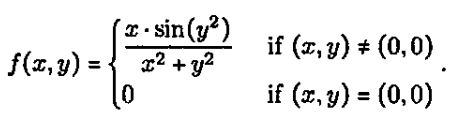Chia-Chung We>試卷(2022/09/19)

# 111 年 - 111 國立臺灣大學轉學生招生考試試題_理工科聯招：微積分(B)#110846

【非選題】
1.

1.

【題組】(a)=__(1)__

【非選題】
2.【題組】

(b)=__(2)__

【非選題】
3.【題組】

(c)=__(3)__

【非選題】
4.
2.

【題組】

(a) Let f(x,y)==__(4)__。

【非選題】
5.【題組】

(b) Let g(x) ==__ (5)__

【非選題】
6.

3. Consider a function f : (-π,π)→R deined byIf f is differentiable on (-π,π), then (a, b) = __(6)__

【非選題】
7.4. Consider the paramettic curvex = 2t2 + 1, y= 4t. Let P be the point (2p2 + 1,4P). The greatest value of p such that the normal to the curve at P passes through (31, -24) is p =__ (7)__

【非選題】
8.

5. Let f(x,y,z)=dt. The linearization of f(x,y,z) at (1.1,0) is L(x,y,z)=__(8)__

【非選題】
9.
6.

【題組】

(a)do=__(9)__

【非選題】
10.【題組】

(b) Let D be the region enclosed by the curve y =and the x-axis. The volume of the solid obtained by revolving D about the x-axis is (10)

【非選題】
11.7. Let f(x, u) = 2x3 - 12xy +y3 +13. Let P= (p，q) be the point on R2 at which the rate of change of f(x,y) in the direction it j is the smallest. Then (p,q) =__ (11)__

【非選題】
12.
8.

【題組】

(a)dydx=_________

【非選題】
13.【題組】

(b) Let R={(x,y)= __(13)__

【非選題】
14.
9.

【題組】

(a) The work done by the force feld F(x, y) =j in moving a particle along a triangular path with vertices (0,0), (1,0), (2,2) counter-clockwisely is__ (14)__

【非選題】
15.【題組】

(b) Let S be part of the cone z =that lies bellow the plane :x + z = 1. ThenxdS=__(15)__

【非選題】
16.【題組】

(c) Let D be a closed surface in R3, oriented outward. The maximum Aux of the vector fieldamong all possible choices of D is __(16)__.

【非選題】
17.

10. The greatest value of p such that the seriesconverges conditionally is p=__(17)__.

【非選題】
18.

1. Consider the function f:R2→ R defined by【題組】 (a) Is f continuous at (0,0)? Justify your answer.

【非選題】
19.【題組】(b) Let u = ai+ bj be a unit vector. Find the directional derivative of f at (0,0) in the direction u. Express your answer in terms of a and b.

【非選題】
20.【題組】(c) Find the direction(s) that f changes the most rapidly at (0,0).

### 懸賞詳解

#### 國三國文上第三次

25.「太陽慢慢地接近山頂，小米園又開始熱鬧了，像辦年貨一樣，成群的小鳥飛來像要飽餐一頓似的。我正要 用力拉動手上的牽引繩，祖父叫住了我：...